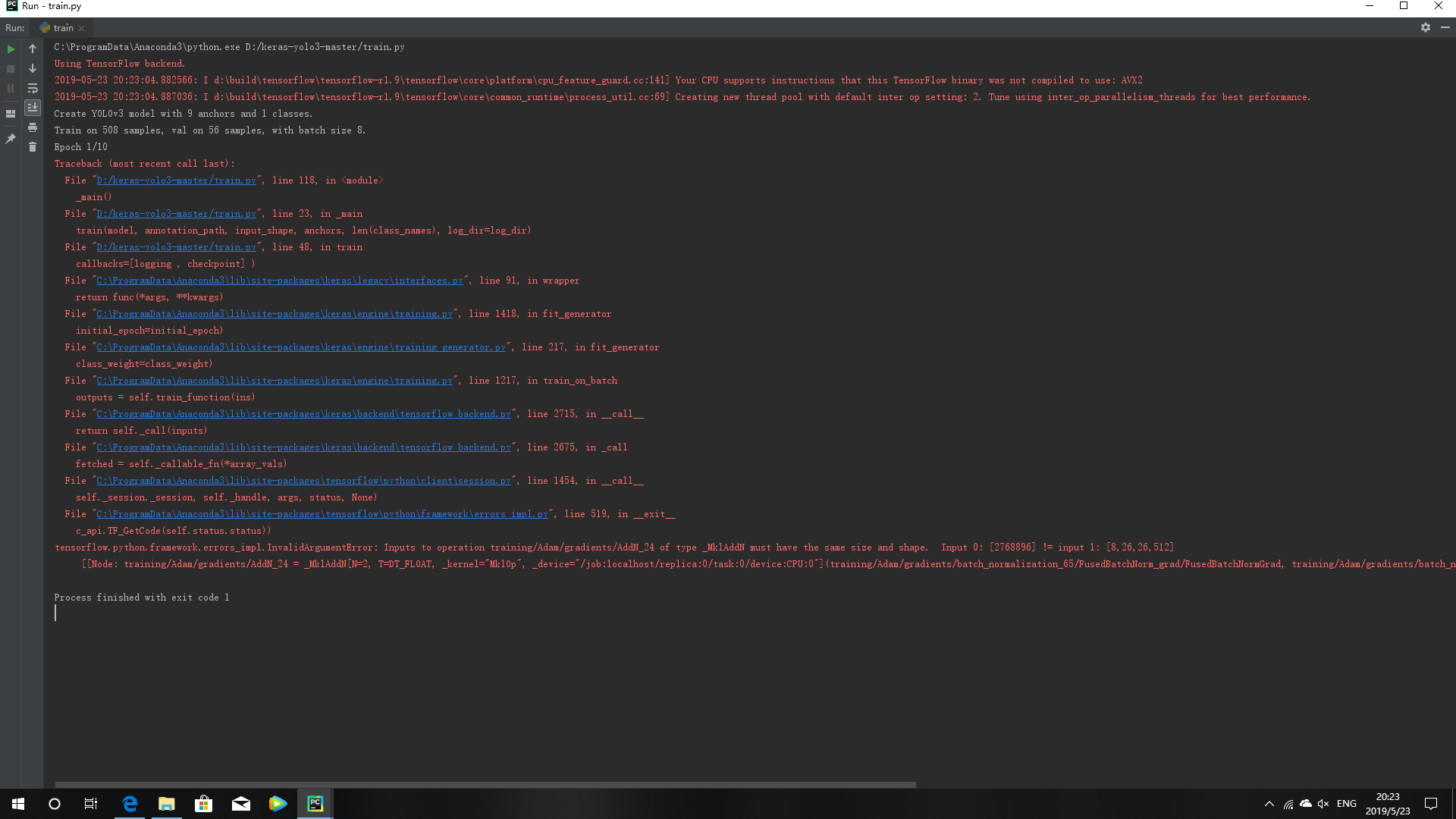``````import numpy as np
import keras.backend as K
from keras.layers import Input, Lambda
from keras.models import Model
from keras.callbacks import TensorBoard, ModelCheckpoint, EarlyStopping
from yolo3.model import preprocess_true_boxes, yolo_body, tiny_yolo_body, yolo_loss
from yolo3.utils import get_random_data

def _main():
annotation_path = 'train.txt'
log_dir = 'logs/000/'
classes_path = 'model_data/voc_classes.txt'
anchors_path = 'model_data/yolo_anchors.txt'
class_names = get_classes(classes_path)
anchors = get_anchors(anchors_path)
input_shape = (416,416) # multiple of 32, hw
model = create_model(input_shape, anchors, len(class_names) )
train(model, annotation_path, input_shape, anchors, len(class_names), log_dir=log_dir)

def train(model, annotation_path, input_shape, anchors, num_classes, log_dir='logs/'):
'yolo_loss': lambda y_true, y_pred: y_pred})
logging = TensorBoard(log_dir=log_dir)
checkpoint = ModelCheckpoint(log_dir + "ep{epoch:03d}-loss{loss:.3f}-val_loss{val_loss:.3f}.h5",
monitor='val_loss', save_weights_only=True, save_best_only=True, period=1)
batch_size = 8
val_split = 0.1
with open(annotation_path) as f:
np.random.shuffle(lines)
num_val = int(len(lines)*val_split)
num_train = len(lines) - num_val
print('Train on {} samples, val on {} samples, with batch size {}.'.format(num_train, num_val, batch_size))

model.fit_generator (
data_generator_wrapper ( lines[:num_train] , batch_size , input_shape , anchors , num_classes ) ,
steps_per_epoch=max ( 1 , num_train // batch_size ) ,
validation_data=data_generator_wrapper (
lines[num_train:] , batch_size , input_shape , anchors , num_classes ) ,
validation_steps=max ( 1 , num_val // batch_size ) ,
epochs=10 ,
initial_epoch=0 ,
callbacks=[logging , checkpoint] )

model.save_weights(log_dir + 'trained_weights.h5')

def get_classes(classes_path):
with open(classes_path) as f:
class_names = [c.strip() for c in class_names]
return class_names

def get_anchors(anchors_path):
with open(anchors_path) as f:
anchors = [float(x) for x in anchors.split(',')]
return np.array(anchors).reshape(-1, 2)

def create_model(input_shape, anchors, num_classes, load_pretrained=False, freeze_body=False,
weights_path='model_data/yolo_weights.h5'):
K.clear_session() # get a new session
h, w = input_shape
image_input = Input(shape=(w, h, 3))
num_anchors = len(anchors)
y_true = [Input(shape=(h//{0:32, 1:16, 2:8}[l], w//{0:32, 1:16, 2:8}[l],
num_anchors//3, num_classes+5)) for l in range(3)]

model_body = yolo_body(image_input, num_anchors//3, num_classes)
print('Create YOLOv3 model with {} anchors and {} classes.'.format(num_anchors, num_classes))

if freeze_body in [1, 2]:
# Do not freeze 3 output layers.
num = (185 , len ( model_body.layers ) - 3)[freeze_body - 1]
for i in range(num):
model_body.layers[i].trainable = False
print('Freeze the first {} layers of total {} layers.'.format(num, len(model_body.layers)))
model_loss = Lambda ( yolo_loss ,
output_shape=(1 ,) , name='yolo_loss',
arguments={'anchors': anchors,
'num_classes': num_classes,
'ignore_thresh': 0.5}
)(model_body.output + y_true)
model = Model(inputs=[model_body.input] + y_true, outputs=model_loss)
return model
def data_generator(annotation_lines, batch_size, input_shape, anchors, num_classes):
n = len(annotation_lines)

i = 0
while True:
image_data = []
box_data = []
for b in range(batch_size):
if i==0:
np.random.shuffle(annotation_lines)
image, box = get_random_data(annotation_lines[i], input_shape, random=True)
image_data.append(image)
box_data.append(box)
i = (i+1) % n
image_data = np.array(image_data)
box_data = np.array(box_data)
y_true = preprocess_true_boxes(box_data, input_shape, anchors, num_classes)
yield [image_data]+y_true, np.zeros(batch_size)

def data_generator_wrapper(annotation_lines, batch_size, input_shape, anchors, num_classes):
n = len(annotation_lines)
if n==0 or batch_size<=0: return None
return data_generator(annotation_lines, batch_size, input_shape, anchors, num_classes)

if __name__ == '__main__':
_main()

``````pycharm中，删除创建的.csv文件失败是什么原因？
pycharm运行mnist_show.py出现如下问题，
pycharm工具中，使用xpath定位元素的时候报错是怎么回事？

mac下pycharm配置Python3.4解释器显示unsupported，提示不支持，请大家帮忙看一下，谢谢

pycharm上面简单的python代码运行报错怎么解决？貌似是pycharm的环境没有配置好，跪求大神指点！

pycharm中.proto文件不能识别

pycharm中如何用tensoflow-gpu运行文件
python3.0import argparse时报错

Pycharm中ui转化.py出现错误，求解决

pycharm 中python 沒有编译报错提示吗
pycharm创建不了工程的问题

pycharm 编译代码之后点了debug 之后不知道该怎么办

pygame的资料已经下载好了，也在环境中安装好了，怎么在pycharm中调用时老是报错？哪位大神教教我，非常感谢！

![图片说明](https://img-ask.csdn.net/upload/201910/11/1570764654_226893.png) ``` import os lst = os.listdir(os.getcwd()) # 获取当前目录下所有的文件名 for c in lst: if os.path.isfile(c) and c.endswith('.py') and c.find("run")== -1: #判断文件名是以.py结尾的，并且去掉run.py文件 print(c) #查看文件 os.system('python {}'.format(c)) #相当于在终端执行文件 python main.py ``` 用这段代码的问题就是，第一个0.py文件运行后，因为是一个死循环的程序，所以后面的文件一直不能运行，而且后面的文件也是死循环的文件。

Python十大装B语法
Python 是一种代表简单思想的语言，其语法相对简单，很容易上手。不过，如果就此小视 Python 语法的精妙和深邃，那就大错特错了。本文精心筛选了最能展现 Python 语法之精妙的十个知识点，并附上详细的实例代码。如能在实战中融会贯通、灵活使用，必将使代码更为精炼、高效，同时也会极大提升代码B格，使之看上去更老练，读起来更优雅。 1. for - else 什么？不是 if 和 else 才

2019年11月中国大陆编程语言排行榜
2019年11月2日，我统计了某招聘网站，获得有效程序员招聘数据9万条。针对招聘信息，提取编程语言关键字，并统计如下： 编程语言比例 rank pl_ percentage 1 java 33.62% 2 c/c++ 16.42% 3 c_sharp 12.82% 4 javascript 12.31% 5 python 7.93% 6 go 7.25% 7

SQL-小白最佳入门sql查询一

“狗屁不通文章生成器”登顶GitHub热榜，分分钟写出万字形式主义大作

"狗屁不通文章生成器"登顶GitHub热榜，分分钟写出万字形式主义大作
GitHub 被誉为全球最大的同性交友网站，……，陪伴我们已经走过 10+ 年时间，它托管了大量的软件代码，同时也承载了程序员无尽的欢乐。 万字申请，废话报告，魔幻形式主义大作怎么写？兄dei，狗屁不通文章生成器了解一下。这个富有灵魂的项目名吸引了众人的目光。项目仅仅诞生一周，便冲上了GitHub趋势榜榜首（Js中文网 -前端进阶资源教程）、是榜首哦

《程序人生》系列-这个程序员只用了20行代码就拿了冠军

8年经验面试官详解 Java 面试秘诀

1.两种思维方式在求职面试中，经常会考察这种问题：北京有多少量特斯拉汽车？某胡同口的煎饼摊一年能卖出多少个煎饼？深圳有多少个产品经理？一辆公交车里能装下多少个乒乓球？一个正常成年人有多少根头发？这类估算问题，被称为费米问题，是以科学家费米命名的。为什么面试会问这种问题呢？这类问题能把两类人清楚地区分出来。一类是具有文科思维的人，擅长赞叹和模糊想象，它主要依靠的是人的第一反应和直觉，比如小孩...

so easy！ 10行代码写个"狗屁不通"文章生成器